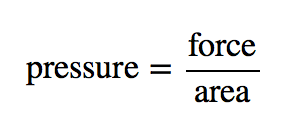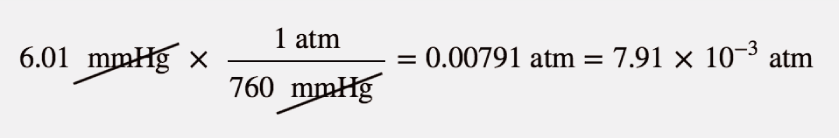Chapter 6. Gases

# Pressure

### Learning Objectives

1. Define pressure.
2. Learn the units of pressure and how to convert between them.

The kinetic theory of gases indicates that gas particles are always in motion and are colliding with other particles and the walls of the container holding them. Although collisions with container walls are elastic (i.e., there is no net energy gain or loss because of the collision), a gas particle does exert a force on the wall during the collision. The accumulation of all these forces distributed over the area of the walls of the container causes something we call pressure. Pressure (P) is defined as the force of all the gas particle/wall collisions divided by the area of the wall:All gases exert pressure; it is one of the fundamental measurable quantities of this phase of matter. Even our atmosphere exerts pressure—in this case, the gas is being “held in” by the earth’s gravity, rather than the gas being in a container. The pressure of the atmosphere is 101,325 Pa.

Pressure has a variety of units. The formal, SI-approved unit of pressure is the pascal (Pa), which is defined as 1 N/m2 (one newton of force over an area of one square meter). However, this is usually too small in magnitude to be useful. A common unit of pressure is the atmosphere (atm), which was originally defined as the average atmospheric pressure at sea level.

However, “average atmospheric pressure at sea level” is difficult to pinpoint because of atmospheric pressure variations. A more reliable and common unit is millimeters of mercury (mmHg), which is the amount of pressure exerted by a column of mercury exactly 1 mm high. An equivalent unit is the torr, which equals 1 mmHg (The torr is named after Evangelista Torricelli, a seventeenth-century Italian scientist who invented the mercury barometer.) With these definitions of pressure, the atmosphere unit is redefined: 1 atm is defined as exactly 760 mmHg, or 760 torr. We thus have the following equivalences:

1 atm = 760 mmHg = 760 torr

We can use these equivalences as with any equivalences—to perform conversions from one unit to another. Relating these to the formal SI unit of pressure, 1 atm = 101,325 Pa.

### Example 1

How many atmospheres are there in 595 torr?

Solution

Using the pressure equivalences, we construct a conversion factor between torr and atmospheres: 1 atm = 760 torr. Thus,Because the numbers in the conversion factor are exact, the number of significant figures in the final answer is determined by the initial value of pressure.

Test Yourself

How many atmospheres are there in 1,022 torr?

Answer

1.345 atm

### Example 2

The atmosphere on Mars is largely CO2 at a pressure of 6.01 mmHg. What is this pressure in atmospheres?

Solution

Use the pressure equivalences to construct the proper conversion factor between millimeters of mercury and atmospheres.At the end, we expressed the answer in scientific notation.

Test Yourself

Atmospheric pressure is low in the eye of a hurricane. In a 1979 hurricane in the Pacific Ocean, a pressure of 0.859 atm was reported inside the eye. What is this pressure in torr?

Answer

652 torr

### Key Takeaways

• Pressure is a force exerted over an area.
• Pressure has several common units that can be converted.

### Exercises

1. Define pressure. What causes it?

2. Define and relate three units of pressure.

3. If a force of 16.7 N is pressed against an area of 2.44 m2, what is the pressure in pascals?

4. If a force of 2,546 N is pressed against an area of 0.0332 m2, what is the pressure in pascals?

5. Explain why the original definition of atmosphere did not work well.

6. What units of pressure are equal to each other?

7. How many atmospheres are in 889 mmHg?

8. How many atmospheres are in 223 torr?

9. How many torr are in 2.443 atm?

10. How many millimeters of mercury are in 0.334 atm?

11. How many millimeters of mercury are in 334 torr?

12. How many torr are in 0.777 mmHg?

13. How many pascals are in 1 torr?

14. A pressure of 0.887 atm equals how many pascals?

Answers

1.

Pressure is force per unit area. It is caused by gas particles hitting the walls of their container.

3.

6.84 Pa

5.

Because the atmospheric pressure at sea level is variable, it is not a consistent unit of pressure.

7.

1.17 atm

9.

1,857 torr

11.

334 mmHg

13.

133 Pa

## LicensePressure by Jessie A. Key is licensed under a Creative Commons Attribution-NonCommercial-ShareAlike 4.0 International License, except where otherwise noted.